1. Modeling the Inflaton Field

The equations of motion derived above (72), (73) describe the evolution of an arbitrary potential V(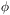) subject only to the constraint that V() conform to the slow roll conditions away from its minimum. As mentioned previously, the conditions for inflation are arbitrary and inflation will occur as long as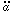> 0. There are three cases that are of particular interest .

• Polynomial Inflation. V()n. This gives a scale factor which behaves quasi-exponentially.For particle theorist n = 2, 4 are most favorable, since they describe renormalizable particle theories .

• Power-law Inflation. V() ~ exp (-sqrt[(16/ n)]/ Mp). This gives a(t) ~ tn. The only requirement being that n > 1.

• Intermediate Inflation. V() ~F, where F = 4(n-1 - 1). This yields a(t) ~ e(t/t0)n.

As an example consider a simple polynomial model with n = 2.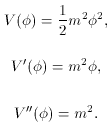The slow roll condition< 1 impllies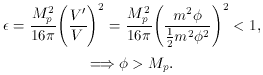In other words, the inflaton field must be larger than the Planck energy. This is actually the value one would expect for the cosmological constant, since the only natural scale at high energy is the Planck scale. However, inflation (as it has been presented) relies on the evolution of a classical field. If the value of the field is in the quantum regime, where the Planck scale lives, inflation can not be treated classically. Fortunately, there are many resolutions to this problem. First, what really matters in the field equations is the potential energy density of the field; namely,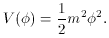Remember that V appeared in the Lagrangian density, thus V is actually a density. From this equation one can see that the magnitude of the potential, and, therefore, the energy scale of the theory, depend on `m' as well as. `m' represents the mass of the inflaton and one resolution to the high value ofis to introduce a small mass for the inflaton; i.e., make `m' small.

Another resolution to this scale problem is to limit considerations to the final part of the evolution of the inflaton. As stated before, the inflaton evolves until= 1 when the inflaton then reheats the universe. The most important consequences of inflation are its resolution of the initial value problems and its predictions about large-scale structure and the cosmic background anisotropies. As we will see most of these phenomena only require analysis of the last moments of `e-foldings' of inflation. E-folding is a way of measuring how the scale factor increases. Since,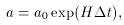one e-folding is defined as the amount of time for a to grow by a factor of e: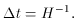It will be shown that only 60 e-foldings are needed to resolve the initial value problems and the scales that are important in determining structure in the universe only depend on modes that are present during these last 60 e-foldings.

However, it must be pointed out that many people find problems with these conditions on. The idea of fine-tuning the mass of the inflaton `m' is certainly unappealing. One of the appealing aspects of inflation was its resolution of the initial value or fine-tuning problems of the Big Bang model. But, now we are again confronted with an initial value problem. This problem can be resolved by addressing inflation in the context of a quantum gravity theory such as superstring theory. I will not pursue such issues in this paper, although the reader is again referred to the eprint archive for recent efforts (27)

27 http://xxx.lanl.gov.